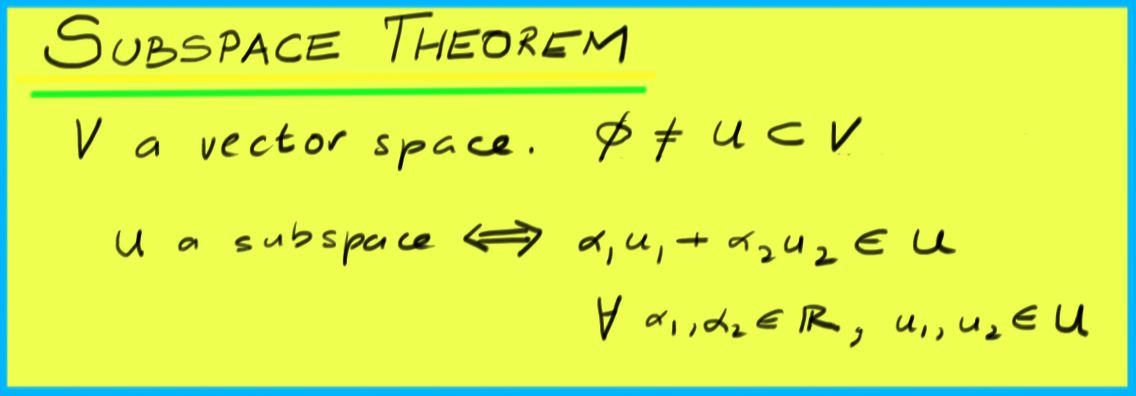$$\newcommand{\id}{\mathrm{id}}$$ $$\newcommand{\Span}{\mathrm{span}}$$ $$\newcommand{\kernel}{\mathrm{null}\,}$$ $$\newcommand{\range}{\mathrm{range}\,}$$ $$\newcommand{\RealPart}{\mathrm{Re}}$$ $$\newcommand{\ImaginaryPart}{\mathrm{Im}}$$ $$\newcommand{\Argument}{\mathrm{Arg}}$$ $$\newcommand{\norm}{\| #1 \|}$$ $$\newcommand{\inner}{\langle #1, #2 \rangle}$$ $$\newcommand{\Span}{\mathrm{span}}$$

9.1: Subspaces

$$\newcommand{\vecs}{\overset { \rightharpoonup} {\mathbf{#1}} }$$

$$\newcommand{\vecd}{\overset{-\!-\!\rightharpoonup}{\vphantom{a}\smash {#1}}}$$

Definition: subspace

We say that a subset $$U$$ of a vector space $$V$$ is a $$\textit{subspace}$$ of $$V$$ if $$U$$ is a vector space under the inherited addition and scalar multiplication operations of $$V$$.

Example 100

Consider a plane $$P$$ in $$\Re^{3}$$ through the origin:

$ax+by+cz=0.$This equation can be expressed as the homogeneous system $$\begin{pmatrix}a & b & c\end{pmatrix}\begin{pmatrix}x\\y\\z\end{pmatrix}=0$$, or $$MX=0$$ with $$M$$ the matrix $$\begin{pmatrix}a & b & c\end{pmatrix}$$. If $$X_{1}$$ and $$X_{2}$$ are both solutions to $$MX=0$$, then, by linearity of matrix multiplication, so is $$\mu X_{1} + \nu X_{2}$$:

$M(\mu X_{1} + \nu X_{2}) = \mu MX_{1} + \nu MX_{2} = 0.$

So $$P$$ is closed under addition and scalar multiplication. Additionally, $$P$$ contains the origin (which can be derived from the above by setting $$\mu=\nu=0$$). All other vector space requirements hold for $$P$$ because they hold for all vectors in $$\Re^{3}$$.Theorem (Subspace Theorem)

Let $$U$$ be a non-empty subset of a vector space $$V$$. Then $$U$$ is a subspace if and only if $$\mu u_{1} + \nu u_{2} \in U$$ for arbitrary $$u_{1}, u_{2}$$ in $$U$$, and arbitrary constants $$\mu, \nu$$.

Proof

One direction of this proof is easy: if $$U$$ is a subspace, then it is a vector space, and so by the additive closure and multiplicative closure properties of vector spaces, it has to be true that $$\mu u_{1} + \nu u_{2} \in U$$ for all $$u_{1},u_{2}$$ in $$U$$ and all constants constants $$\mu, \nu$$.

The other direction is almost as easy: we need to show that if $$\mu u_{1} + \nu u_{2} \in U$$ for all $$u_{1}, u_{2}$$ in $$U$$ and all constants $$\mu, \nu$$, then $$U$$ is a vector space. That is, we need to show that the ten properties of vector spaces are satisfied. We know that the additive closure and multiplicative closure properties are satisfied. All of the other eight properties is true in $$U$$ because it is true in $$V$$.

$$\square$$

Note that the requirements of the subspace theorem are often referred to as "closure''.

We can use this theorem to check if a set is a vector space. That is, if we have some set $$U$$ of vectors that come from some bigger vector space $$V$$, to check if $$U$$ itself forms a smaller vector space we need check only two things:

1. If we add any two vectors in $$U$$, do we end up with a vector in $$U$$?
2. If we multiply any vector in $$U$$ by any constant, do we end up with a vector in $$U$$?

If the answer to both of these questions is yes, then $$U$$ is a vector space. If not, $$U$$ is not a vector space.